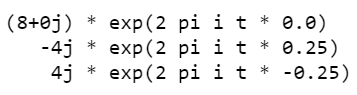GeeksforGeeks App
Open AppBrowser
Continue

# How to extract frequency associated with fft values in Python?

In this article, we will find out the extract the values of frequency from an FFT.  We can obtain the magnitude of frequency from a set of complex numbers obtained after performing FFT i.e Fast Fourier Transform in Python. The frequency can be obtained by calculating the magnitude of the complex number. So simple ab(x) on each of those complex numbers should return the frequency.

### Required methods:

In order to extract frequency associated with fft values we will be using the fft.fft() and fft.fftfreq() methods of numpy module.

• numpy.fft.fft(): It calculates the single-dimensional n-point DFT i.e. Discrete Fourier Transform with an optimized FFT i.e Fast Fourier Transform algorithm.

Syntax: numpy.fft.fft(a, axis=-1)

Parameters:

• a: Input array can be complex.
• axis: Axis over which to compute the FFT. If not given, the last axis is used.

Returns: The truncated or zero-padded input, transformed along the axis indicated by axis, or the last one if the axis is not specified.

• numpy.fft.fftfreq(): It computes the frequencies associated with the coefficients.

Syntax: numpy.fft.fftfreq(n, d=1.0)

• n: Window length.
• d: Sample spacing (inverse of the sampling rate). Defaults to 1.

Returns: Array of length n containing the sample frequencies.

### Step-by-step Approach:

Step 1: Import required modules.

## Python3

 `# import module``import` `numpy as np`

Step 2: Create an array using a NumPy.

## Python3

 `# assign data``x ``=` `np.array([``1``,``2``,``1``,``0``,``1``,``2``,``1``,``0``])`

Step 3: A signal x defined in the time domain of length N, sampled at a constant interval dt, its DFT W(here specifically W = np.fft.fft(x)), whose elements are sampled on the frequency axis with a sample rate dw. (A DFT converts a list of N complex numbers to a list of N complex numbers)

## Python3

 `# compute DFT with optimized FFT``w ``=` `np.fft.fft(x)`

Step 4: The np.fft.fftfreq() method tells you the frequencies associated with the coefficients.

## Python3

 `# compute frequency associated``# with coefficients``freqs ``=` `np.fft.fftfreq(``len``(x))`

Step 5: Extract frequency associated with fft values.

## Python3

 `# extract frequencies associated with FFT values``for` `coef, freq ``in` `zip``(w, freqs):``    ``if` `coef:``        ``print``(``'{c:>6} * exp(2 pi i t * {f})'``.``format``(c``=``coef,``                                                    ``f``=``freq))`

Below is the complete program based in the above approach:

## Python3

 `# import required modules``import` `numpy as np`` ` `# assign data``x ``=` `np.array([``1``, ``2``, ``1``, ``0``, ``1``, ``2``, ``1``, ``0``])`` ` `# compute DFT with optimized FFT``w ``=` `np.fft.fft(x)`` ` `# compute frequency associated``# with coefficients``freqs ``=` `np.fft.fftfreq(``len``(x))`` ` `# extract frequencies associated with FFT values``for` `coef, freq ``in` `zip``(w, freqs):``    ``if` `coef:``        ``print``(``'{c:>6} * exp(2 pi i t * {f})'``.``format``(c``=``coef,``                                                    ``f``=``freq))`

Output:My Personal Notes arrow_drop_up